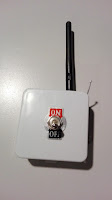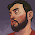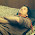## Sunday, January 10, 2016

### ESP8266 JammingThis weekend I finally managed to make time to play around with some ESP8266 based boards I had laying in my garage. I wanted to try and use them standalone, i.e., without any other micro controller like an Arduino or a RPI.
Also, for those of you that don't know my mindset, I was obviously wondering about the raw packet injection capability of the thing! Obviously...

Well, using Arduino IDE 1.6.5 and the additional board manager to program the ESP8266 chip, I made a small program to spam the aether with random beacon frames. It's mostly harmless, but it shows packet injection is working just fine.

I couldn't resist to make a small portable gadget just for the kicks:WiFi Beacon Jam
(I know that powering the thing with a 9V battery is not a very smart thing to do, I made do with what I had in my garage. It's basically just the ESP8266, an external antenna and a battery)

It has only two functions: on and off. :)
Here's the result in my phone WiFi scanner, seems like a christmas tree:

As I said, this is mostly harmless. It's not a *real* jammer. But I can easily imagine pretty interesting times ahead with these little chips.
Possibilities are, as they should be, limitless!

Anyway, it was a fun weekend project.
You can fetch the code at GitHub here (warning: lazy code ahead)!

#### 17 comments:

1.How can we use pre-defined SSID?
Can you show some example?

1.This comment has been removed by the author.

2.#include < ESP8266WiFi.h >

extern "C" {
#include "user_interface.h"
}

byte channel;

uint16_t beaconPacket(uint8_t* output, uint8_t channel, uint8_t* mac, String& ssid){
*(output++) = 0x80;
*(output++) = 0x00;
*(output++) = 0x00;
*(output++) = 0x00;

*(output++) = 0xFF; *(output++) = 0xFF; *(output++) = 0xFF; // DA
*(output++) = 0xFF; *(output++) = 0xFF; *(output++) = 0xFF;
for (uint8_t i=0; i<6; i++) *(output++) = mac[i];           // SA
for (uint8_t i=0; i<6; i++) *(output++) = mac[i];           // BSS ID

*(output++) = 0xC0; *(output++) = 0x6C;                     // Seq-ctl

*(output++) = 0x83; *(output++) = 0x51; *(output++) = 0xF7; *(output++) = 0x8F; // timestamp
*(output++) = 0x0F; *(output++) = 0x00; *(output++) = 0x00; *(output++) = 0x00;

*(output++) = 0x64; *(output++) = 0x00;                     // Beacon interval
*(output++) = 0x01; *(output++) = 0x04;                     // Capability info  0x01 0x04

uint16_t ssid_amount = ssid.length();
*(output++) = 0; *(output++) = ssid_amount;                 // 0 = SSID, length = ssid length

for (uint16_t i=0; i < ssid_amount; i++){
*(output++) = ssid[i];
}

*(output++) = 0x01; *(output++) = 0x08;                     // 1 = supported rates, length = 8
*(output++) = 0x82; *(output++) = 0x84; *(output++) = 0x8B; *(output++) = 0x96;
*(output++) = 0x24; *(output++) = 0x30; *(output++) = 0x48; *(output++) = 0x6C;

*(output++) = 0x03; *(output++) = 0x01; *(output++) = channel; // 3 = DSPS/channel, length = 1

uint16_t returnValue = 51 +ssid_amount;
return returnValue;
}

void setup() {
delay(500);
wifi_set_opmode(STATION_MODE);
wifi_promiscuous_enable(1);
}

void loop() {
channel = random(1,12);
wifi_set_channel(channel);

uint8_t beaconMAC;
beaconMAC = random(256);
beaconMAC = random(256);
beaconMAC = random(256);
beaconMAC = random(256);
beaconMAC = random(256);
beaconMAC = random(256);

String ssid = "Test SSID";

uint8_t  beaconData;
uint16_t packetSize = beaconPacket(beaconData, channel, beaconMAC, ssid);

wifi_send_pkt_freedom(beaconData, packetSize, 0);
wifi_send_pkt_freedom(beaconData, packetSize, 0);
wifi_send_pkt_freedom(beaconData, packetSize, 0);

delay(1);
}

2.okey i found solution how to use pre-defined SSID but i have no idea how to extend them to more that 6 characters.
why help ?

1.You can find a complete explanation here: http://mrncciew.com/2014/10/08/802-11-mgmt-beacon-frame/

3.offset 36 and offset 37 are the length of the SSID

1.36 = Element ID, 0 means ssid element
37 = Element length, in this case: ssid length
38-43 = the ssid string
44 = Element ID, 1 means supported rates
45 = Element length, 8 supported rates
46-53 = list of supported rates
54 = Element ID, 3 means wifi channel
55 = Element length, 1 byte channel
56 = wifi channel

See page 5:
http://research.ijcaonline.org/ctngc/number3/ctngc1027.pdf
And here:
https://mrncciew.com/2014/10/08/802-11-mgmt-beacon-frame/
"Element ID is 0 for the SSID IE. SSID could have maximum of 32 characters."

4.offset 36 and offset 37 are the length of the SSID

5.Cool stuff. Kudos for keeping it simple and sweet. This is going to drive my WiFi monitoring team mad.... Houston we have a problem new access points popping up all the time. Oh the sweet madness...

Lol seriously this has some interesting applications though.

6.you've got 2 small k in your alfa string.
I love the lazy way to create the ssid

7.How do you set the 802.11 data rate? This method transmits data at the slowest rate of 1 Mbps which completely saturates radio air time.

8.I suppose you can use function 'wifi_set_user_sup_rate' or 'wifi_set_user_fixed_rate'

Take a look at the SDK Programming Guide here:
https://github.com/OLIMEX/ESP8266/tree/master/IoT%20Firmware/document/English

1.Thanks a lot! This worked great!

9.Good project thx

10.i can`t get the wifi_send_pkt_freedom to work on my IDE...

11.i can`t get the wifi_send_pkt_freedom to work on my IDE...

12.i had the following erro.

sketch\Attack.cpp: In member function 'bool Attack::send()':

Attack.cpp:109: error: 'wifi_send_pkt_freedom' was not declared in this scope

if (wifi_send_pkt_freedom(packet, packetSize, 0) == -1) {

^

exit status 1
'wifi_send_pkt_freedom' was not declared in this scope

what i can do